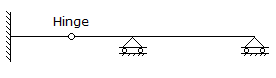# Civil Engineering - UPSC Civil Service Exam Questions - Discussion

35.

The degree of indeterminacy of the beam given in the given figure is[A]. zero [B]. one [C]. two [D]. three

Explanation:

No answer description available for this question.

 Penny said: (Apr 10, 2017) I think Option C is correct.

 Dev said: (Sep 16, 2017) B is correct.

 Mahesh said: (Jan 2, 2018) Answer must be B.

 Dolly said: (Mar 25, 2018) B is the correct answer.

 Phani said: (Apr 30, 2018) Please explain it.

 Dolly said: (Aug 22, 2018) Indeterminacy = no.of unknown forces - no. of equilibrium equation. No.of unknown forces = 3(for fixed) +2(one for each roller support). Indeterminacy = 5 - 4(3(no. of equilibrium equation) + 1 for internal hinge). = 1.

 Umesh Jit said: (Sep 15, 2018) Great explanation, Thanks @Dolly.

 Rajeev said: (Jul 5, 2019) B should be the correct answer.

 Prasi said: (Jan 11, 2020) Degree of indeterminacy = r-3+ no of hinges. = 5-3+1 = 3. Or degree= 3m + r -3j + n. = 3(3) +5 -3(4) +1 = 3.

 Nirmal said: (May 7, 2020) Unknown reactions = fix(3)+rollers(2) + internal hinge (1 horizontal reaction) = 6. D. O. Indeterminacy =6-3=3 =>(D).